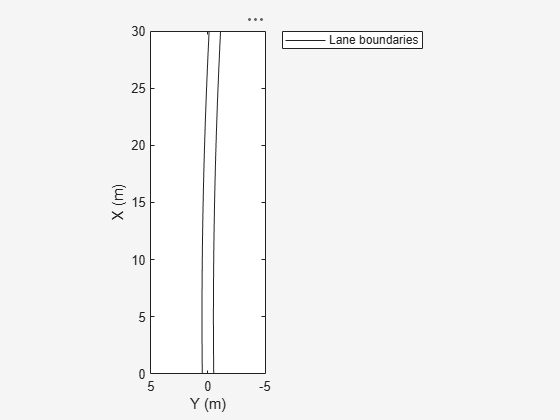# parabolicLaneBoundary

Parabolic lane boundary model

## Description

The `parabolicLaneBoundary` object contains information about a parabolic lane boundary model.

## Creation

To generate parabolic lane boundary models that fit a set of boundary points and an approximate width, use the `findParabolicLaneBoundaries` function. If you already know your parabolic parameters, create lane boundary models by using the `parabolicLaneBoundary` function (described here).

### Syntax

``boundaries = parabolicLaneBoundary(parabolicParameters)``

### Description

example

````boundaries = parabolicLaneBoundary(parabolicParameters)` creates an array of parabolic lane boundary models from an array of ```[A B C]``` parameters for the parabolic equation y = Ax2 + Bx + C. Points within the lane boundary models are in world coordinates.```

### Input Arguments

expand all

Coefficients for parabolic models of the form y = Ax2 + Bx + C, specified as an `[A B C]` real-valued vector or as a matrix of `[A B C]` values. Each row of `parabolicParameters` describes a separate parabolic lane boundary model.

## Properties

expand all

Coefficients for a parabolic model of the form y = Ax2 + Bx + C, specified as a real-valued vector of the form `[A B C]`.

Type of lane boundary, specified as a `LaneBoundaryType` enumeration. Supported lane boundary types are:

• `Unmarked`

• `Solid`

• `Dashed`

• `BottsDots`

• `DoubleSolid`

Lane boundary objects always return `BoundaryType` as type `Solid`. Update these types to match the types of the lanes that are being fitted. To update a lane boundary type, use the `LaneBoundaryType.BoundaryType` syntax. For example, this code sample shows how to update the first output lane boundary to type `BottsDots`:

```boundaries(1) = LaneBoundaryType.BottsDots; ```

Strength of the boundary model, specified as a real scalar. `Strength` is the ratio of the number of unique x-axis locations on the boundary to the length of the boundary specified by the `XExtent` property. A solid line without any breaks has a higher strength than a dotted line that has breaks along the full length of the boundary.

Length of the boundary along the x-axis, specified as a real-valued vector of the form `[minX maxX]` that describes the minimum and maximum x-axis locations.

## Object Functions

 `computeBoundaryModel` Obtain y-coordinates of lane boundaries given x-coordinates

## Examples

collapse all

Create left-lane and right-lane parabolic boundary models.

```llane = parabolicLaneBoundary([-0.001 0.01 0.5]); rlane = parabolicLaneBoundary([-0.001 0.01 -0.5]);```

Create a bird's-eye plot and lane boundary plotter. Plot the lane boundaries.

```bep = birdsEyePlot('XLimits',[0 30],'YLimits',[-5 5]); lbPlotter = laneBoundaryPlotter(bep,'DisplayName','Lane boundaries'); plotLaneBoundary(lbPlotter, [llane rlane]);```Find lanes in an image by using parabolic lane boundary models. Overlay the identified lanes on the original image and on a bird's-eye-view transformation of the image.

Load an image of a road with lanes. The image was obtained from a camera sensor mounted on the front of a vehicle.

`I = imread('road.png');`

Transform the image into a bird's-eye-view image by using a preconfigured sensor object. This object models the sensor that captured the original image.

```bevSensor = load('birdsEyeConfig'); birdsEyeImage = transformImage(bevSensor.birdsEyeConfig,I); imshow(birdsEyeImage)```Set the approximate lane marker width in world units (meters).

`approxBoundaryWidth = 0.25;`

Detect lane features and display them as a black-and-white image.

```birdsEyeBW = segmentLaneMarkerRidge(im2gray(birdsEyeImage), ... bevSensor.birdsEyeConfig,approxBoundaryWidth); imshow(birdsEyeBW)```Obtain the image coordinates corresponding to the lane candidate positions. The `find` function returns pixel indices that correspond to the candidate lane positions. By convention, the order of the image coordinates is always reversed relative to the pixel indices. For more information about image coordinates, see Coordinate Systems.

Obtain the corresponding lane boundary points in vehicle coordinates by using the `imageToVehicle` function.

```[imageY,imageX] = find(birdsEyeBW); xyBoundaryPoints = imageToVehicle(bevSensor.birdsEyeConfig,[imageX,imageY]);```

Find lane boundaries in the image by using the `findParabolicLaneBoundaries` function. By default, the function returns a maximum of two lane boundaries. The boundaries are stored in an array of `parabolicLaneBoundary` objects.

`boundaries = findParabolicLaneBoundaries(xyBoundaryPoints,approxBoundaryWidth);`

Use `insertLaneBoundary` to overlay the lanes on the original image. The `XPoints` vector represents the lane points, in meters, that are within range of the ego vehicle's sensor. Specify the lanes in different colors. By default, lanes are yellow.

```XPoints = 3:30; figure sensor = bevSensor.birdsEyeConfig.Sensor; lanesI = insertLaneBoundary(I,boundaries(1),sensor,XPoints); lanesI = insertLaneBoundary(lanesI,boundaries(2),sensor,XPoints,'Color','green'); imshow(lanesI)```View the lanes in the bird's-eye-view image.

```figure BEconfig = bevSensor.birdsEyeConfig; lanesBEI = insertLaneBoundary(birdsEyeImage,boundaries(1),BEconfig,XPoints); lanesBEI = insertLaneBoundary(lanesBEI,boundaries(2),BEconfig,XPoints,'Color','green'); imshow(lanesBEI)```## Version History

Introduced in R2017a